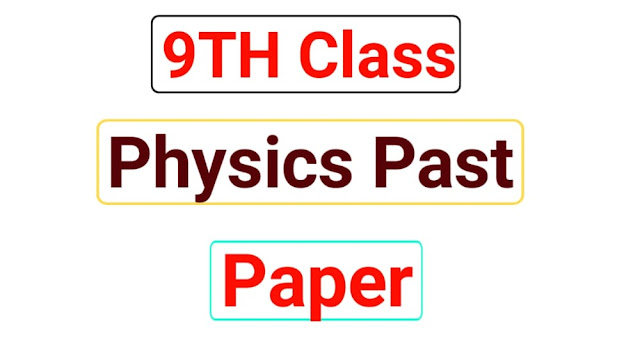# 9th Class Physics Past Paper English Medium9th Class Physics Past Paper 2021 English Medium

### 9th Class Physics Past Paper English Medium

• What is meant by base quantities give one example?
• Express 4800000w in kilo and megawatt by using prefixes?
• How least count of Vernier calipers can be calculated?
• Differentiate between scalars and vectors?
• What is meant by rest and motion?
• Define velocity write down its mathematical formula?
• Why does a passenger move outward when a bus takes a turn?
• Define friction and limit friction?

 For More Updates Join Whatsapp Group Posted Date 16 September 2021 Book Code Physics Degree Matric Post Title 9th Class Physics Past Paper 2021 English Medium Current/Past Past Paper 2021 Mid/Final Final Paper Year 2021 Last Post 9TH Class Chemistry Past Paper

#### When a body is said to be in equilibrium?What is a rigid body? Give an example of a moving body that is in equilibrium? What is meant by the force of gravitation? Why is the law of gravitation important to us? What is the difference between g and G? Define energy give two types of mechanical energy?What is meant by the efficiency of a system?

• Define density and elasticity?
• Why does atmosphere pressure change with height?
• Define tensile strain write down its formula?
• What is meant by thermal equilibrium?
• Define heat capacity write down its unit?
• Differentiate between conduction and convection?
• What is meant by convection currents?
• What is meant by the transfer of heat?

 We Also Recommended

9th Class Physics Past Paper 2021 English Medium

### LONG QUESTIONS

• State law of conservation of momentum explain it with the example of balls?
• A car has a velocity of 10ms-1 it accelerates at 0.2ms-2 for half a minute find the distance traveled during this time and the final velocity of the car?
• Define equilibrium state and explain the first condition for equilibrium?
• A 500 g stone is thrown up with a velocity of 15ms-1 find its?
• P.E at its maximum height?
• K.E when it hits the ground?
• Define the coefficient of linear thermal expansion and prove that @= ΔL/L.ΔT
• A student presses her palm by her thumb with a force of 75 N what would be the pressure under her thumb having a contact area of 1.5cm2

Students we hope you read to complete this post and we are happy to read complete our struggle but we daily upload this type of post you can check our website. Our aim is to create the latest post and publish important study solutions for student's help so that students can easily read and learn from our website. Please share 9th Class Physics Past Paper English Medium on WhatsApp groups or Facebook groups. Students this is our most struggle to write and then publish it by spending our much of time please support us and read all our posts